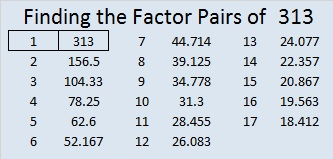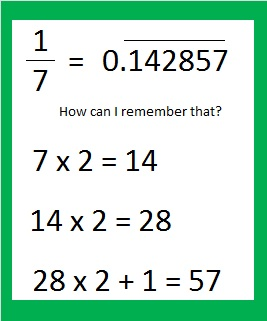# 313 and Memorizing One Seventh

• 313 is a prime number.
• Prime factorization: 313 is prime.
• The exponent of prime number 313 is 1. Adding 1 to that exponent we get (1 + 1) = 2. Therefore 313 has exactly 2 factors.
• Factors of 313: 1, 313
• Factor pairs: 313 = 1 x 313
• 313 has no square factors that allow its square root to be simplified. √313 ≈ 17.692How do we know that 313 is a prime number? If 313 were not a prime number, then it would be divisible by at least one prime number less than or equal to √313 ≈ 17.692. Since 313 cannot be divided evenly by 2, 3, 5, 7, 11, 13, or 17, we know that 313 is a prime number.

Question: Liam set a goal to read all of the Harry Potter books. What percentage of the books had he read once he finished the first book? Answer: 1/7 ≈ 14.2857%, which looks like a much more complicated percentage than it really is. 1/7 is a repeating decimal that isn’t too difficult to remember. Here’s how I remember it:A famous approximation for pi is 22/7 = 3 1/7. If you have 1/7 memorized then you can also easily remember that pi is approximately equal to 3.142857. (It’s true that 355/113 ≈ 3.141592 is a better approximation since it’s accurate to six decimal places while 22/7 is accurate to only two. You can remember 355/113 by thinking 113355 and putting the first three digits in the denominator and the last three digits in the numerator.)

Here’s some other questions to consider: What percentage of the names of the seven dwarfs can you remember? What decimal amount of the seven wonders of the world can you name? What percentage of days last week did you exercise? How many of the seven deadly sins….

The decimals for 2/7, 3/7, 4/7, 5/7, and 6/7 are also easy and use the same very cool pattern, 142857, but each fraction starts with a different digit. Here’s how to remember each of those decimals, too:Go ahead and make somebody think you’re a human calculator today!

## One thought on “313 and Memorizing One Seventh”

1.Joseph Nebus

I was showing off to my love the Ancient Egyptian method of multiplication and division by doubling numbers (part of complaining about long division) and we picked some numbers arbitrarily. After a bit of work my love noticed the six-digit repeating decimal that things petered out to, and I realized that we’d picked numbers that, yeah, summoned the old 1/7th pattern. I felt embarrassed I didn’t notice the only factor the numbers we picked didn’t have in common was a 7.

This site uses Akismet to reduce spam. Learn how your comment data is processed.